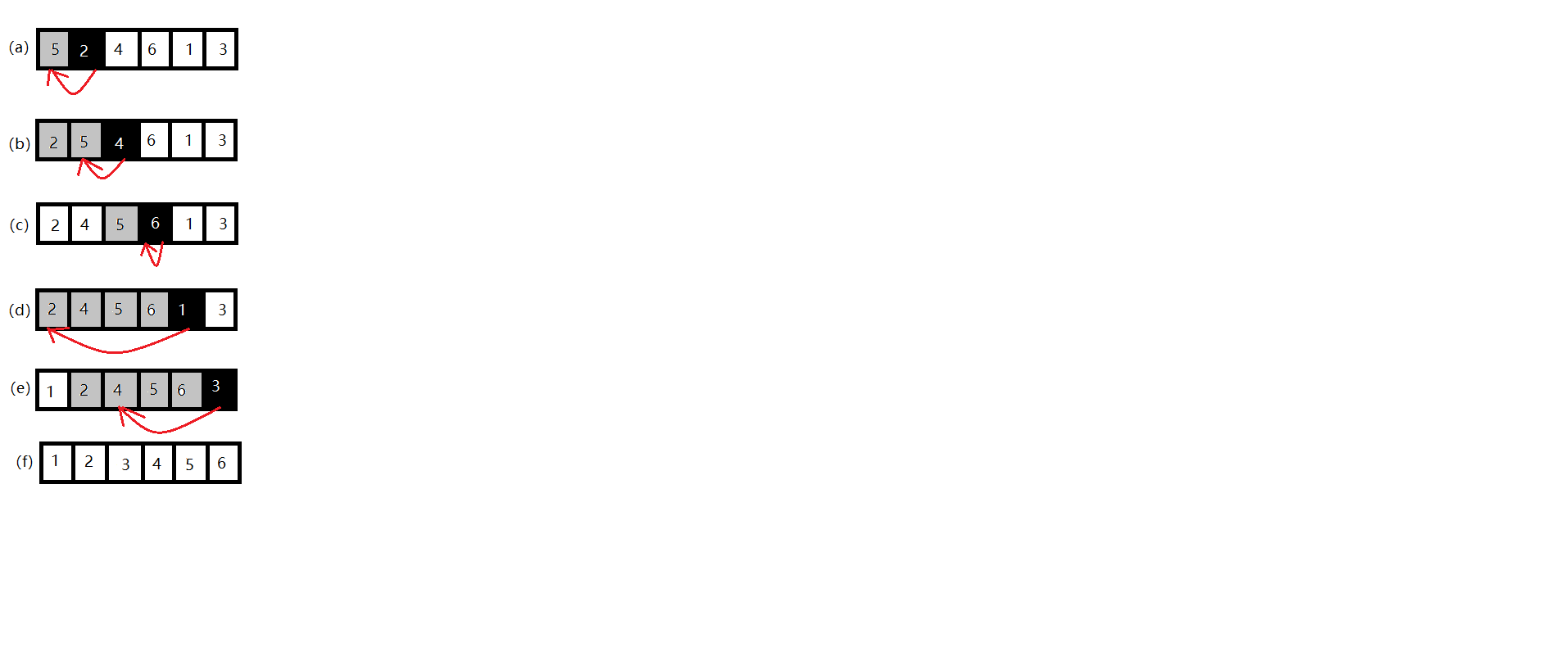# 数据结构与算法（一）插入排序

## 1.1 排序问题

从整个待排序列中选出一个元素插入到已经有序的子序列中去，得到一个有序的、元素加一的子序列，直到整个序列的待插入元素为0，则整个序列全部有序。

## 1.2 实现伪代码

INSERTION-SORT(A)
for 2 to A.length
key = A[j];
// Insert A[j] into the sorted sequence A[1..j-1]
i = j - 1;
while i > 0 and A[j] > key
A[i + 1] = A[i]
i = i - 1
A[i + 1] = key

## 1.3 步骤解析## 1.3 算法分析

INSERTION-SORT(A) 代价 次数
for 2 to A.length $c_1$ n
key = A[j]; $c_2$ n - 1
// Insert A[j] into the sorted sequence A[1…j-1]  0 n - 1
i = j - 1;  $c_4$ n - 1
while i > 0 and A[j] > key  $c_5$ $\sum_{j=2}^n$$t_j​$
A[i + 1] = A[i] $c_6$ $\sum_{j=2}^n$($t_j​$ - 1)
i = i - 1 $c_7$ $\sum_{j=2}^n$($t_j$ - 1)
A[i + 1] = key $c_8$ n - 1

• $t_j$表示对那个值j第5行执行while循环的次数
• 当一个forwhile循环按通常的方式（即由于循环头中的测试）退出时，执行测试的次数比执行循环体的次数多1
• 注释是不可执行的语句，所以不需要时间

T(n) = $c_1​$n + $c_2​$(n - 1) + $c_4​$(n - 1) + $c_5​$$\sum_{j=2}^n​$$t_j​$ + $c_6​$ $\sum_{j=2}^n​$($t_j​$ - 1) + $c_7​$$\sum_{j=2}^n​$($t_j​$ - 1) + $c_8​$(n - 1)

1.3.1 最佳情况

$T(n) = c_1$n + $c_2$(n - 1) + $c_4$(n - 1) + $c_5$(n - 1) + $c_8$(n - 1)

$= （c_1​+ c_2​ + c_4​ + c_5​ + c_8​）n - （c_2 + c_4 + c_5 + c_8​）$

$= \Theta(n)​$

1.3.2 最坏情况

T(n) = c_1 n + $c_2$(n-1) + $c_4$(n-1) + $c_5$( $\frac{n(n+1)}{2}-1$) + $c_6(\frac{n(n-1)}{2})$ + $c_7$($\frac{n(n-1)}{2}$) + $c_8$(n-1)

= $(\frac{c_5}{2} + \frac{c_6}{2} +\frac{c_7}{2} )$$n^2$ + $(c_1 + c_2 +c_4 + \frac{c_5}{2} -\frac{c_6}{2} \frac{c_7}{2} + c_8)$$n-$($c_2+c_4+c_5+c_8$)

= $\Theta(n^2)$

1.3.3两种情况的区别

• 算法的最坏情况提供了一个上界，知道了上界能确保算法不需要更长的时间。
• 对于某些算法最坏情况经常出现。
• “平均情况”往往与最坏情况大致一样差。

©️2019 CSDN 皮肤主题: 深蓝海洋 设计师: CSDN官方博客